Impact Factor 3.560 | CiteScore 3.1
More on impact ›

# Frontiers in Physics## REVIEW article

Front. Phys., 15 February 2021 | https://doi.org/10.3389/fphy.2020.613392

# Cooperative Dynamics in the Fiber Bundle Model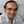Bikas K. Chakrabarti1,2,Soumyajyoti Biswas3 andSrutarshi Pradhan4*
• 1Saha Institute of Nuclear Physics, Kolkata, India
• 2S. N. Bose National Centre for Basic Sciences, Kolkata, India
• 3SRM University-AP, Andhra Pradesh, India
• 4PoreLab, Department of Physics, Norwegian University of Science and Technology, Trondheim, Norway

We discuss the cooperative failure dynamics in the fiber bundle model where the individual elements or fibers are Hookean springs that have identical spring constants but different breaking strengths. When the bundle is stressed or strained, especially in the equal-load-sharing scheme, the load supported by the failed fiber gets shared equally by the rest of the surviving fibers. This mean-field-type statistical feature (absence of fluctuations) in the load-sharing mechanism helped major analytical developments in the study of breaking dynamics in the model and precise comparisons with simulation results. We intend to present a brief review on these developments.

## 1 Introduction

Fiber bundle model (FBM) has been used widely for studying the fracture and failure  of composite materials under external loading. The simplicity of the model allows us to achieve analytic solutions  to an extent that is not possible in any other fracture models. For these very reasons, FBM is widely used as a model of breakdown that extends beyond disordered solids. In fact, FBM was first introduced in connection with textile engineering . Physicists took interest in it recently to explore the critical failure dynamics and avalanche phenomena during such stress-induced failures . Apart from the classical fracture–failure in composites, FBM has been used successfully for studying noise-induced (creep/fatigue) failure  where a fixed load is applied on the system and external noise triggers the failure of elements. Furthermore, it was used as a model for other geophysical phenomena, such as snow avalanche , land slides [16, 17], biological materials , or even earthquakes . In this review article, we concentrate only on the cooperative dynamical aspects in FBM.

F. T. Peirce, a textile engineer, introduced the fiber bundle model  in 1926 to study the strength of cotton yarn. Later, in 1945, Daniels discussed some static behavior of such a bundle  and the model was brought to the attention of physicists in 1989 by Sornette  who started analyzing the failure process. Even though FBM was designed initially as a model for the fracture or failure of a set of parallel elements (fibers), having different breaking thresholds, with a collective load-sharing scheme, the failure dynamics in the model shows all the attributes of the critical phenomena and the associated phase transition. It seems, due to the usefulness and richness, FBM plays the same role (in the field of fracture) as the Ising model in magnetism .

In FBM, a number of parallel Hookean springs or fibers are clamped between two horizontal platforms (Figure 1). The breaking strengths of the springs or fibers are different. When the load per fiber (stress) exceeds a fiber’s own threshold, it fails. The load it carries has to be shared by the surviving fibers. If the lower platform deforms under loading while the upper platform remains rigid, fibers in the neighborhood of the just-failed fiber will absorb more of the load compared to fibers sitting further away, and this arrangement is called the local-load-sharing (LLS) scheme [23, 24]. If both the platforms are rigid, the load has to be equally distributed among all the surviving fibers, which is called the equal-load-sharing (ELS) scheme. Intermediate load redistribution schemes are also studied (see, e.g., ), where a part of the load is shared locally within a few fibers and the rest is shared globally among all the fibers.

FIGURE 1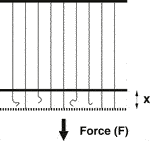FIGURE 1. A cartoon of the fiber bundle model where a macroscopically large number (N) of Hookean springs, with identical spring constants but different breaking thresholds, hang parallelly from an upper rigid bar and a load/force F is applied at the lower horizontal rigid bar (not allowing any local deformation of the bar and consequent local stress concentration). If any spring fails at any time, the (extra) load is shared by the surviving fibers at that time. In the equal-load-sharing scheme, considered here, this extra load is shared equally by all the surviving fibers (x denoting the strain of the surviving fibers).

How does cooperative dynamics set in? In the case of ELS, all the intact fibers carry the load equally. When a fiber fails, the stress level increases on the remaining fibers and that can trigger more fiber failures (successive failure). As long as the initial load is low, the successive failures of the fibers remain small, and though the strain (stretch) of the bundle grows with increasing stress (load), the bundle as a whole does not fail. Once the initial load reaches a “critical” value, determined by the fiber strength distribution, the successive failures become global (catastrophic) and the bundle collapses.

We arrange this review article as follows: In the short introduction (Section 1), we elaborate the concept of the fiber bundle model and its evolution as a fracture model. Section 2 deals with the equal-load-sharing FBM where we demonstrate the dynamic behavior of FBM with evolution dynamics and their solutions. Analytic results are compared with numerical simulations in this section. In Section 3, we discuss noise-induced failure dynamics in FBM through theory, simulation, and real data analysis. The self-organizing mechanism in FBM is discussed in Section 4. We reserve Section 5 for discussions on some works that would help to understand the cooperative dynamics in FBM. Finally, we have a short Summary and Conclusion section (Section 6) at the end.

## 2 Equal Load Sharing FBM

We consider an FBM having N parallel fibers placed between two rigid bars. Each fiber follows Hook’s law with a force f to the stretch value x as $f=\kappa x$, where κ is the spring constant. To make things simpler, we consider $\kappa =1$ for all the fibers. Each fiber has a particular strength threshold value and if the stretch x exceeds this threshold, the fiber fails irreversibly. We are interested in the equal-load-sharing (ELS) mode (the bars are rigid), and by construction of the model, the applied load has to be shared equally by the intact fibers.

Other than the analytical treatment of the model, several aspects of the model are also explored numerically. The implementation of the model, particularly in the equal load sharing version we discuss here, is straightforward. The load is initially applied to each fiber equally. The fibers having failure thresholds less than the applied load are irreversibly broken. The load carried by those fibers is redistributed equally among the remaining fibers, which can cause further breaking. The redistribution continues until no new fibers are breaking. The external load is held constant during the whole redistribution process. This is due to the separation of time scales of externally applied loading rate and the internal (elastic) relaxation processes within materials. After the end of each redistribution cycle, the external load is further increased to continue the dynamics. This process continues until the entire system is broken. The critical strength, avalanche statistics, and other critical exponents are calculated from this dynamics, which, as we will see, match well with the analytical results.

### 2.1 Fiber Strength Distributions

The fiber strength thresholds are drawn from a probability density of $p\left(x\right)$. The corresponding cumulative probability is

$P(x)=∫0xp(y)dy.(1)$

The most used threshold distributions are uniform and Weibull distributions (see Figure 2) in the FBM literature.

FIGURE 2

For a uniform distribution, we can write

$p(x)=1;P(x)=x,(2)$

where, the range of function is between 0 and 1. The cumulative Weibull distribution has the form:

$P(x)=1−exp(−xk),(3)$

where, k is the shape parameter or the Weibull index. The corresponding probability distribution takes the form:

$p(x)=kxk−1exp(−xk).(4)$

The shapes of the uniform and Weibull distributions are shown in Figure 2. The range of definition is between 0 and $\infty$.

### 2.2 The Critical Values

When we stretch the bundle by applying a force, the fibers fail according to their thresholds, the weakest first, then the next weakest, and so on. If ${N}_{f}$ fibers have failed at a stretch value of x, the force on the bundle is

$F=(N−Nf)x=N(1−P(x))x,(5)$

as $\kappa =1$. The normalized force $\left(F/N\right)$ versus the stretch x curve looks like a parabola (Figure 3).

FIGURE 3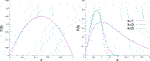FIGURE 3. Normalized force $\left(F/N\right)$ against extension x for a fiber bundle with uniform $\left({x}_{c}=0.5\right)$ and Weibull ${x}_{c}=1,{\left(1/3\right)}^{1/3},{\left(1/5\right)}^{1/5}$ for $k=1,3,5$, respectively, distributions of strengths (thresholds).

It is obvious that the maximum of the force value is the strength of the bundle, and the corresponding stretch value $\left({x}_{c}\right)$ is called the critical stretch beyond which the bundle collapses. Therefore, we can define two distinct phases of the system: stable phase for $0 and unstable phase for $x>{x}_{c}$.

The critical stretch value can be obtained easily by setting $\text{d}F\left(x\right)/\text{d}x=0$:

$1−xcp(xc)−P(xc)=0.(6)$

1. Uniform threshold distribution

Substituting the $p\left({x}_{c}\right)$ and $P\left({x}_{c}\right)$ values for uniform distribution, we obtain

$xc=(12).(7)$

Now inserting the ${x}_{c}$ value in the force expression (Eq. 5), we get

$FcN=14;(8)$

which is the critical strength of the bundle (Figure 3).

2. Weibull threshold distribution

In the case of Weibull distribution, at the force-maximum, by inserting the $P\left(x\right)$ and $p\left(x\right)$ values into the expression (Eq. 6), we obtain

$exp(−xck)−(xckxck−1⁡exp(−xck))=0.(9)$

One can get the critical stretch value

$xc=k−1/k;(10)$

and the corresponding critical force value

$FcN=k−1/k⁡exp(−1k).(11)$

For $k=1$, ${x}_{c}=1.0$ and $\left({F}_{c}/N\right)=\left(1/e\right)$ (Figure 3).

Now, we will discuss how the load or stress can be applied on the bundle. In the FBM literature, the most common loading mechanism discussed [26, 27] is the -weakest-link-failure mechanism of loading. This loading process ensures a separation in time scales between external loading and internal stress redistribution. This is equivalent to a quasi-static approach, and noise/fluctuation in the threshold distribution influences the breaking dynamics as well as the avalanche statistics.

### 2.4 The Cooperative Dynamics

We are going to discuss the cooperative dynamical behavior of the breaking processes for the bundle loaded by fixed amount per step (following the formulations in the References [1, 2, 4, 8, 26, 27, 31]).

Let us assume that an external force F is applied to the fiber bundle. The stress on the bundle (the external load per fiber) is

$σ=F/N.(12)$

Let us call ${N}_{t}$ to be the number of surviving fibers after t steps in the stress redistribution cycle, with ${N}_{0}=N$.

Now, the effective stress becomes

$σt=Nσ/Nt.(13)$

Therefore, $NP\left(N\sigma /{N}_{t}\right)$ of fibers will fail in the first stress redistribution cycle. The number of intact fibers in the next cycle will be

$Nt+1=N−NP(Nσ/Nt).(14)$

Using ${n}_{t}={N}_{t}/N$, Eq. 14 takes the form of a recursion relation,

$nt+1=1−P(σ/nt),(15)$

with σ as the control parameter and ${n}_{0}=1$ as the start value.

The character of an iterative dynamics is determined by its fixed points (denoted by *) where a dynamical variable remains exactly at the same value it had in the previous step of the dynamics. In other words, a fixed point is a value (of a dynamical variable) that is mapped onto itself by the iteration. The dynamics stops or it becomes locked at the fixed point.

One can find out the possible fixed points $n\text{*}$ of Eq 15, which satisfy

$n∗=1−P(σ/n∗),(16)$

and the solutions of the breaking dynamics at the fixed point.

### 2.5 The Critical Exponents

If we consider that the fiber strengths follow uniform distribution, the recursion relation can be written as

$nt+1=1−σ/nt,(17)$

Consequently, at the fixed point, the relation assumes a simple form

$(n∗)2−n∗+σ=0,(18)$

with solution

$n*=12±(σc−σ)1/2.(19)$

Here the critical stress value is ${\sigma }_{c}=\left(1/4\right)$, beyond which the bundle collapses completely. In Eq. 19, the upper sign gives ${n}^{\ast }>{n}_{c}$, which corresponds to a stable fixed point. From this solution, it is easy to derive the order parameter, susceptibility, and relaxation time (all defined below).

The fixed-point solution gives the critical value $\left(\sigma ={\sigma }_{c}\right)$.

$nc∗=12.(20)$

Therefore, the fixed-point solution can be presented as

$n∗(σ)−nc∗∝(σc−σ)β, β=12.(21)$

Clearly, $n\text{*}\left(\sigma \right)-{n}_{c}^{\text{*}}$ can be considered like an order parameter, which shows a clear transition from nonzero to zero value at ${\sigma }_{c}$.

The susceptibility is defined as $\chi =-d{n}^{\ast }/\text{d}\sigma$ and the fixed-point solution gives

$χ∝(σc−σ)−γ, γ=12;(22)$

which follows a power law and diverges at the critical point ${\sigma }_{c}$.

The dynamical approach very near a fixed point is very interesting, and this can be investigated by expanding the differences ${n}_{t}-n\text{*}$ around the fixed point. In the case of uniform distribution, the recursion relation (Eq. 17), gives

$nt+1−n∗=σn∗−σnt=σntn∗(nt−n∗)≃σn∗2(nt−n∗).(23)$

Clearly, the fixed point is approached with exponentially decreasing steps:

$nt−n*∝e−t/τ,(24)$

where τ is a relaxation parameter, dependent on stress value:

$τ=1/ln(n∗2/σ)=1/ln[(12+14−σ)2/σ].(25)$

At the critical stress, $\sigma ={\sigma }_{c}=1/4$, the argument of the logarithm is 1 and apparently τ is infinite. As the critical stress is approached for $\sigma \to {\sigma }_{c}$.

This divergence clearly shows the character of the breaking dynamics, that is, it becomes very slow at the critical point.

### 2.6 Universal Behavior

The recursion relation and the fixed point solutions demonstrated the dynamic critical behavior for the uniform distribution of the breaking thresholds. Now the question arises—how general the results are? The universality of the cooperative breaking dynamics can be verified by considering a different distribution of fiber strengths. We are now going to examine the situation for a linearly increasing distribution (Figure 4) within the interval $\left(0,1\right)$,

$p(x)={2x,0≤x≤1,0,x>1.(27)$

FIGURE 4

From the force–stretch relationship, the average force per fiber is

$F(x)/N={x(1−x2),0≤x≤1,0,x>1.(28)$

Therefore, the critical point is

$σc=233.(29)$

In this case, the breaking dynamics can be written as a recursion relation:

$nt+1=1−(σ/nt)2,(30)$

and the fixed-point equation is

$(n∗)3−(n∗)2+σ2=0,(31)$

that is, a cubic equation in $n\text{*}$. Clearly, there are three solutions of $n\text{*}$ for a value of σ. At the critical stress value, ${\sigma }_{c}=2/3\sqrt{3}$, the only acceptable solution of Eq. 31 is

$nc∗=23.(32)$

We want to investigate the breaking dynamics in the neighborhood of the critical point. Therefore, we insert $n=2/3+\left(n-{n}_{c}\right)$ into (Eq. 30), with the result

$427−(n−nc)2−(n−nc)3=σ2=(233+σ−σc)2=427+433(σ−σc)+(σ−σc)2.(33)$

$(n−nc)2=433(σc−σ).(34)$

Obviously, for $\sigma \le {\sigma }_{c}$ the order parameter behaves as

$n(σ)−nc∝(σc−σ)β, β=12,(35)$

in accordance with (Eq. 21). The susceptibility $\chi =-dn/\text{d}\sigma$ gives

$χ∝(σc−σ)−γ, γ=12.(36)$

We can also discuss how the stable fixed point is approached from below. From Eq. 30, one can write, around the fixed point,

$nt+1−n∗=σ2n∗2−σ2nt2=σ2n∗2nt2 (nt2−n∗2)≃(nt−n∗)2σ2n∗3.(37)$

The approach is clearly exponential,

The argument of the logarithm becomes 1 exactly at the critical point; therefore, τ diverges when the critical state is approached. The nature of such divergence assumes the same form,

$τ∝(σc−σ)−θ, θ=12,(39)$

which is similar to the model with a uniform fiber strength distribution, Eq. 26.

We can now conclude that the ELS FBM with a linearly increasing fiber strength distribution possesses the same critical power laws as the ELS FBM with a uniform fiber strength distribution. This confirms that the critical properties of cooperative breaking dynamics are universal. A general treatment for verifying universality in ELS FBM can be found in Reference .

### 2.7 Two-Sided Critical Divergence

When a fixed amount of load is applied on the system, the iterative breaking process ends with one of the two possible end results. Either the whole bundle collapses, or an equilibrium situation is reached where intact fibers can hold/support the applied load/stress. Thus, the final fate of the bundle depends on whether the external stress σ on the bundle is postcritical $\left(\sigma >{\sigma }_{c}\right)$, precritical $\left(\sigma <{\sigma }_{c}\right)$, or critical $\left(\sigma ={\sigma }_{c}\right)$. It is interesting to know how the breaking dynamics is approaching the critical point (failure point) from below (precritical) and above (postcritical) stress values.

In the case of uniform fiber strength distribution when the external stress approaches the critical value of ${\sigma }_{c}=1/4$ from a higher value, that is, in the postcritical region, the number of necessary iterations needed for the whole system to break increases as the critical point is approached. Close to the critical point, the number of iterations shows a square root divergence :

$tf≃12π(σ−σc)−1/2.(40)$

Similarly, in the precritical region, when the external stress approaches the critical value of ${\sigma }_{c}=1/4$ from below, the number of iterations has again a square root divergence  (for uniform distribution) close to the critical point:

$tf=14ln(N)(σc−σ)−1/2.(41)$

The only difference is that, in precritical case, the amplitude of the square root divergence has a system-size-dependence, which is absent in the postcritical case.

We can conclude that in ELS FBM, the breaking dynamics shows a two-sided critical divergence in terms of the number of iteration steps needed to reach critical points from below (precritical) and above (postcritical) (Figure 5). The theoretical details of the exact solutions can be found in References [8, 26].

FIGURE 5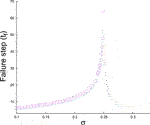FIGURE 5. Postcritical and precritical relaxation: Numerical data are for a bundle with $N={10}^{6}$ fibers having uniform threshold distribution and averages are taken over ${10}^{5}$ samples. Lines are showing theoretical estimates.

The number of fibers (S) breaking between two successive stable conditions of the fiber bundle is called an avalanche. The distribution of the avalanche sizes $P\left(S\right)$ shows a power-law tail for the large S limit , which is a sign of the criticality discussed above. This is experimentally widely observed for driven disordered systems in general  and for quasi-brittle/ductile fracture in particular. While the details of the avalanche dynamics seen in the fiber bundle model with quasi-static load increase has been discussed elsewhere in this special issue , here we briefly describe the avalanche dynamics for fixed amount load increase, that is, when the system is in a stable condition, a fixed amount of load δ is added, which restarts the dynamics. As before, the number of fibers breaking until the system reaches the next stable state constitutes an avalanche. Clearly, this type of avalanche is a result of the cooperative breaking dynamics, and it is not arising due to any fluctuations in stress levels or in fiber strength distribution. We will describe below how to calculate theoretically the distribution of such avalanches.

The load curve, in terms of the threshold values, can be written as

$F(x)=Nx(1−x).(42)$

For the uniform threshold distribution in $\left(0,1\right)$ (see Eq. 5). The load increases between 0 and $N/4$ with an increment of δ. Therefore, the values of the load are $m\delta$, with $m=0,1,2,\dots ,N/4\delta$. The threshold value for load $m\delta$ can be obtained from (Eq. 42 as

$xm=12(1−1−4mδ/N).(43)$

The average number of fibers broken due to the increase of load from $m\delta$ to $\left(m+1\right)\delta$ is

$S=Ndxmdm=δ1−4mδ/N.(44)$

The number of avalanches of size between S and $S+\text{d}S$ is obtained from the corresponding interval of the variable m, that is, $P\left(S\right)\text{d}S=\text{d}m$. From the equation above, we have

$dSdm=2S3(Nδ).(45)$

Therefore, the avalanche size distribution is given by

$P(S)=dmdS=12NδS−3, forS≥δ.(46)$

Indeed, it is possible to show 26] that for an arbitrary threshold distribution, $p\left(x\right)$, the large S asymptotic limits of the avalanche size distribution is

$P(S)∼CS−3,(47)$

with $C=\left(N\delta p{\left({x}_{c}\right)}^{2}/2p\left(x\right)+{x}_{c}{p}^{\prime }\left({x}_{c}\right)\right)$, with the mild assumption that the load curve has a generic parabolic form with a critical point.

## 3 Noise-Induced Failure in FBM

So far we have discussed the classical stress-induced failure of fibers without the presence of noise. A noise-induced failure scheme for the fiber bundle model can be formulated [13, 14, 33] for which the cooperative failure dynamics can be solved analytically.

As in the previous sections, we consider a bundle of N parallel fibers clamped between two rigid bars. A load or force $\left(F=\sigma N\right)$ is applied on the bundle. The fibers have different strength thresholds (x), and there is a critical strength ${\sigma }_{c}$  for the whole bundle, so that the bundle does not fail completely for stress $\sigma \le {\sigma }_{c}$, but it fails immediately for $\sigma >{\sigma }_{c}$. Now we introduce noise (T) in the system and assume that each fiber having the strength of ${x}_{i}$ has a finite probability ${P}_{f}\left(\sigma ,T\right)$ of failure at any stress σ induced by a noise T:

$Pf(σ,T)={Cexp[−1T(xiσ−1)],0≤σ≤xi,1,σ>xi.(48)$

Here C is a prefactor. ${P}_{f}\left(\sigma ,T\right)$ increases as T increases and for a fixed value of T and ${\sigma }_{c}$, as we increase σ, the bundle breaks more rapidly. The motivation behind (Eq. 48) comes from the time-dependent behavior or the so-called creep behavior of materials, observed in real systems [10, 26]. It is obvious that the strength of elements/fibers degrades in time due to external influences like moisture, temperature, etc.

Such a noise-induced failure scheme will produce two different failure regimes depending on the stress and noise levels—continuous breaking regime and intermittent breaking regime. In the continuous breaking regime, we can calculate the failure time (step) as a function of stress and noise values. However, in the intermittent breaking regime, one can define the waiting time between two consecutive failure phases.

The phase boundary can be determined through a mean-field argument that at $\sigma ={\sigma }_{0}$, at least one fiber must break to trigger the continuous fracturing process. After this single failure, the applied load has to be redistributed on the intact fibers (due to ELS) and the effective stress will surely increase (more than ${\sigma }_{0}$), which in turn enhances failure probability for all the intact fibers. Following this logic, in the case of a homogeneous bundle where all the fibers have identical strength, ${x}_{i}=1$ (and ${\sigma }_{c}=1$), at the phase boundary $N{P}_{f}\left({\sigma }_{0},T\right)\ge 1$ giving

$N exp[−1T(1σ0−1)]≥1.(49)$

which finally gives

$σ0≥11−T⁡log(1/N).(50)$

In absence of noise, when $T=0$, the above equation gives ${\sigma }_{0}=1={\sigma }_{c}$, which is consistent with the static FBM results . This analytic estimate overlaps with the data obtained from simulation (Figure 6). It shows that the continuous and intermittent fracturing regimes are separated by a well-defined phase boundary, which depends on both the stress level and the noise level .

FIGURE 6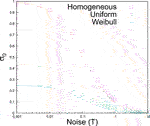FIGURE 6. Phase boundary (${\sigma }_{0}$ vs. T plot) for three different types of fiber strength distributions with $N=20000$. Data points are simulation results and solid lines are analytic estimates (Eq. 50) based on mean-field arguments.

In the case of heterogeneous FBMs where fibers have different strength thresholds, keeping in mind that in absence of noise T, we should always get ${\sigma }_{0}={\sigma }_{c}$, one can make a conjecture that

$σ0≥σc1−T⁡log(1/N).(51)$

The numerical data for the heterogeneous cases (Figure 6) having uniform and Weibull-type fiber strength distributions supports the conjecture well .

Identification of such a phase boundary has important consequences in material-fracturing and in other similar fracture-breakdown phenomena. During material/rock fracturing, acoustic emission (AE) measurements can record the burst or avalanche events in terms of AE amplitude and AE energy . Therefore, AE data could reveal the correct rupture-phase of a material body under stress. Once a system enters into continuous rupture phase, the system collapse must be imminent. Thus, identification of the rupture phase can guide us to visualize the final fate of a system. It can also help to stop system collapse, if it is possible to withdraw external stress in time before the system enters into continuous rupture phase.

We will now discuss cooperative dynamics in both these regimes in the following sub-sections.

### 3.1 Continuous Breaking Regime

In the continuous breaking regime, one can describe the breaking dynamics in an FBM through a recursion relation . Let us consider a homogeneous bundle having N fibers with exactly the same strength thresholds of 1; therefore, critical (or failure) strength of the bundle is ${\sigma }_{c}=1$. Now, we consider a noise-induced failure probability for breaking of each fiber in the continuous regime:

$Pf(σ,T)={σσcexp[−1T(xiσ−1)],0≤σ≤xi,1,σ>xi.(52)$

As all the fibers are identical, ${x}_{i}=1={\sigma }_{c}$. The prefactor is a function of stress level σ, and this is a careful choice to get a solution of the recursive dynamics, which we will describe below.

We denote the fraction of total fibers that remain intact at time (step) t by ${n}_{t}$ and the breaking dynamics can be written as

$nt+1=nt[1−Pf(σnt,T)].(53)$

In the continuum limit, the above recursion relation can be presented in a differential form

$−dndt=σσcexp[−1T(σcσn−1)].(54)$

Giving the failure time

$tf=T⁡exp(−1T)[exp(σcσT)−1].(55)$

The simulation result shows (Figure 7) the exact agreement with this theoretical estimate.

FIGURE 7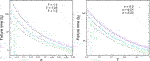FIGURE 7. Failure time versus σ (left) and versus T (right) for a homogeneous bundle having identical fibers with a strength of 1 (${\sigma }_{c}=1$ as well). The data are for simulations over a single realization with a system size of $N=1000000$, and the solid lines are the theoretical estimates following (Eq. 55).

In the case of heterogeneous bundles where fibers have distributed strengths, the failure times seem to follow another form :

$tf=T⁡exp(−1T)[exp(σcσT+1T)−1].(56)$

This form was obtained through a trial and error approach. It is extremely difficult (as of now) to write the recursion relation for noise-induced failure dynamics in the case of heterogeneous systems. The simulation results have been compared with the formula above, and the agreement (Figure 8) is quite satisfactory .

FIGURE 8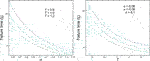FIGURE 8. Failure time versus σ (left) and versus T (right) for bundles having uniform strength distributions. The data are for simulations over 1000 realizations with a system size of $N={10}^{6}$, and the solid lines are the theoretical estimates following (Eq. 56).

### 3.2 Intermittent Regime

As we discussed before, in the intermittent fracturing phase, simultaneous breaking events (avalanches) are separated by waiting times $\left({t}_{W}\right)$ of different magnitudes. The waiting time distribution can be fitted with a Gamma distribution  for both homogeneous and heterogeneous bundles

$D(tW)∝exp(−tW/a)/tW1−γ(57)$

where $\gamma =0.15$ for homogeneous case and $\gamma =0.26$ for heterogeneous cases (Figure 9). Here a is a measure of the extent of the power law regime, and it seems that the power law exponent does not change with the variation of σ, T, and N .

FIGURE 9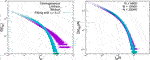FIGURE 9. Left: The simulation results for the waiting time distributions for three different types of fiber strength distributions, with $N=20000$. All the curves can be fitted with the Gamma form $\mathrm{exp}\left(-{t}_{W}/a\right)/{t}_{W}^{1-\gamma }$, where $\gamma =0.15$ is for the homogeneous case and $\gamma =0.26$ is for uniform and Weibull distributions. Right: we show the data collapse of the waiting time distributions with system sizes for uniform fiber strength distribution.

In the waiting time distributions, the power law part dominates for small ${t}_{W}$ values and exponential law dominates for bigger ${t}_{W}$ values. The inherent global load sharing nature is responsible for the power law part of the Gamma distribution, as power law usually comes from a long range cooperative mechanism [6, 35, 36]. The exponential part of the Gamma distribution is contributed by the noise-induced failure factor ${P}_{f}\left(\sigma ,T\right)$. For large ${t}_{W}$ values, one can eventually treat the failures to be independent. If P indicates the noise-induced failure probability within ${t}_{W}$, then the probability $D\left({t}_{W}\right)=A{\left(1-P\right)}^{{t}_{W}N}\sim \mathrm{exp}\left(-P{t}_{W}N\right)$, where A is a constant. The normalization of $D\left({t}_{W}\right)$ requires $A\sim N.$ Though for smaller values of ${t}_{W}$, one cannot ignore the correlations between successive failures (responsible for the power law part in $D\left({t}_{W}\right)$), the exponential scaling behavior for $D\left({t}_{W}\right)$ can be easily obtained from the above. As shown in the inset of Figure 9, the plot of $D\left({t}_{W}\right)/N$ against ${t}_{W}N$ gives good data collapse for different N values. Such a data collapse indicates the robustness of the Gamma function form. It is not clear yet whether the Gamma-type distribution is a direct consequence of the failure probability function (Eq. 48). It needs more investigations with various other types of possibilities for Eq. 48.

Apparently, the modeling scheme for noise-induced rupture process is not limited to any particular system, rather it is a general approach and perhaps it can model more complex situations like rupture-driven earthquakes. In literature, we can find evidences of stress-localization around fracture/fault lines in an active seismic-zone. Also, there are several factors that can help rupture evolution, like friction, plasticity, fluid migration, spatial heterogeneities, chemical reactions, etc. To some extent, such stress redistribution/localization can be taken into account through a proper load sharing scheme and a noise term (T) can in principle represent the combined effect of all other factors.

To compare the waiting time results of the model system with real data, the California earthquake catalog from 1984 to 2002  has been analyzed  to study the statistics of waiting times  between earthquake events. First, a cutoff (${m}_{c}$) has been set in the earthquake magnitude, so that all earthquake events above this cutoff magnitude will be considered for the analysis. The distribution of waiting times shows similar variation for different cutoff values. It seems  waiting time distributions for all the data sets follow a Gamma distribution :

$D(tW)∝exp(−tW/a)/tW(1−γ);(58)$

with same γ ($\simeq$ 0.1) and different a values for different cutoff levels: $a=30,120,500,2000$, respectively, for ${m}_{c}=2.5,3.0,3.5,4.0$ (see Figure 10).

FIGURE 10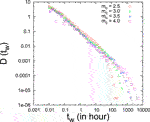FIGURE 10. Gamma-fitting (dotted lines) to the waiting time distributions in California catalog (1984–2002).

The similarities in waiting time statistics and scaling forms suggest that slowly driven (noise-induced) fracturing process and earthquake dynamics (stick-slip mechanism) perhaps have some common origin.

## 4 Interface Propagation in the Fiber Bundles: Self-Organization and Depinning Transition

So far we have considered FBM versions that are globally loaded, that is, all the fibers in the system are loaded equally from the initial time, and the load remains equal on each surviving fiber, given that the load sharing is equal. This necessarily implies that the damage or failures in the system could occur at any point; indeed, there is no notion of distance in this form of the model.

However, in fracture dynamics, particularly in the mode-1 variant of it, a front could propagate in the direction transverse to that of the loading. A fracture front necessarily implies damage localization within a region with a lower dimension than that of the system, that is, a front-line in two dimensions or a front surface in a three-dimensional system. Indeed, front propagation driven through a disordered medium is not limited to fracture; it also happens in the vortex lines in superconductors , magnetic domain walls in magnetic materials with impurities , contact line dynamics in wetting , and so on.

FIGURE 11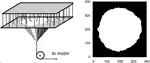FIGURE 11. A schematic representation of locally loaded fiber bundle model and the resulting interface propagation. From .

As the damage perimeter increases, so does the number of fibers on that perimeter. This implies that for an avalanche, the load per fiber will decrease along the damage boundary. But due to a further increase in the load, this value will subsequently increase, initiating another avalanche. In the steady state, the load per fiber value will fluctuate around a constant and the system is said to have reached a self-organized state. In this state, the failure of fibers in the process of avalanches has a scale-free size distribution, which suggests that it is a self-organized critical (SOC) state (where external drive and dissipation balance and the critical point becomes an attractive fixed point ).

The steady-state value of the load per fiber and the corresponding avalanche size distribution can be calculated for a variant of this model where the load redistribution is uniform along the entire damage boundary, that is, every fiber along the damage boundary gets the same fraction of load in a redistribution process. We discuss this for the Weibull distribution below, but this is true for other distributions as well.

The Weibull distribution in its general form can be written as

$Wα,β(x)=αβxα−1e−βxα,(59)$

where α and β are the two parameters. We can consider the particular case when $\alpha =2$ and $\beta =1$. The failure threshold of a fiber is greater than x with a probability that is proportional to ${\int }_{x}^{\infty }x\prime {e}^{-x{\prime }^{2}}\text{d}x\prime \sim {e}^{-{x}^{2}}$. Given that the probability density function for force is uniform, the probability of a fiber having a load between x and $x+\text{d}x$ is ${e}^{-{x}^{2}}P\left(x\right)\text{d}x$, with $P\left(x\right)=c$ (unnormalized). The normalization gives $c{\int }_{0}^{\infty }{e}^{-{x}^{2}}\text{d}x=1$, implying $c=2/\sqrt{\pi }$. Hence, the normalized probability density function for the load on the surviving fibers is

$Dσ(x)=2πe−x2.(60)$

Similarly, the probability that the load is lower than x is proportional to x. Using the form for threshold distribution $\left(\sim x{e}^{-{x}^{2}}\right)$, the probability density function for the threshold distribution of the survived fibers becomes

$Dth(x)=4πx2e−x2.(61)$

Both of these functions are in good agreement with numerical simulations. Also, the saturation value of the average load per fiber can be calculated as

$∫0∞xDσ(x)dx=2π∫0∞xe−x2dx=1π,(62)$

which is again in good agreement with simulations.

The size distribution of avalanches is a power law with the exponent value close to $3/2$ (see Figure 12), which is in agreement with the scaling prediction of avalanche size distributions in SOC models for the mean field. The distribution of the avalanche duration, that is, the number of redistribution steps for an avalanche, is a power law with an exponent value close to $2.00±0.01$, which is again in agreement with the scaling predictions of the SOC models in mean field.

FIGURE 12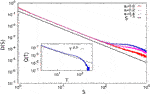FIGURE 12. The avalanche size distributions are plotted for zero and finite lower cutoffs for Model II. The distribution function is a power law with an exponent value of $1.50±0.01$, which is also our estimate from scaling arguments. Inset: The distribution of avalanche duration is plotted for Model II. This also shows a power law decay with an exponent value of $2.00±0.01$. From .

For estimating the avalanche size exponent, it can be assumed that the average load per fiber on the damage boundary has a distribution, which is Gaussian around its mean: $P\left(\sigma \right)\sim {e}^{-{\left(\sigma -{\sigma }_{c}\right)}^{2}/\delta \sigma }$. Hence, from a dimensional analysis, mean-squared fluctuation is $\delta \sigma \sim {\left(\sigma -{\sigma }_{c}\right)}^{2}$. Also, the avalanche size S scales as ${\left(\delta \sigma \right)}^{-1}$ , as it may be viewed as the number of broken fibers after a load increase of $\delta \sigma$. This gives

$(σ−σc)∼S−1/2.(63)$

The probability of an avalanche being of the size between S and $S+\text{d}S$ is $D\left(S\right)\text{d}S$. Now, the deviation from the critical point scales  with the cumulative size of all avalanches up to that point; giving $\left(\sigma -{\sigma }_{c}\right)\sim {\int }_{S}^{\infty }D\left(S\right)\text{d}S$. If we take $D\left(S\right)\sim {S}^{-\gamma }$, then

$(σ−σc)∼S1−γ.(64)$

By comparing Eqs. 63 and 64, we have $\gamma =3/2$. So, the probability density function for the avalanche size becomes $D\left(S\right)\sim {S}^{-3/2}$, which fits well with simulation results (Figure 12).

## 5 Some Related Works on the Dynamics of FBM

In this section, we would like to bring attention to some related works on the dynamics of FBM which, we believe, may be regarded as essential reading in this field.

As we have discussed in detail in the earlier sections, there has been considerable progress in characterizing the failure dynamics in the fiber bundle model through tools describing critical phenomena. One crucial step toward that direction is to identify the universality class of the model. That often needs a coarse grained description of the model, writing down the free energy form suited for the dynamics and then identifying the symmetries and consequently the universality class. One such step was done in Ref.  by writing down a mesoscopic description of the ELS-FBM. By specifically, writing the time evolution of the order parameter $n\left(\sigma \right)-{n}_{c}=\eta$ and the driving field (stress increase) as $J={\sigma }_{c}-\sigma$, the dynamics is described by

$∂η∂t=−η2+J.(65)$

Writing in terms of the density of intact fibers n,

$∂n∂t=λn(1−n)−σ,(66)$

with $\lambda =1$. This equation has a particle-hole symmetry for zero external field $\sigma =0$; hence, it is generally expected to be in the CDP or compact domain growth universality class of non-equilibrium phase transition . Although done for the ELS version, this approach of relating fiber bundle model dynamics to nonequilibrium critical phenomena through a Langevin equation could provide useful insights into more realistic versions.

Among other attempts to relate fracture and in particular FBM dynamics with different universality classes, a relatively less explored route is that of the hydrodynamics of turbulence. The analogy between the velocity fluctuation in turbulence and surface roughness due to fracture have been explored before . However, given that FBM is able to provide a reasonably consistent picture for fracture dynamics, its association with hydrodynamics of fracture is a crucial question. In Ref. , the relation between the Kolmogorov energy dispersion in turbulence and avalanche dynamics in the FBM was explored. Specifically, the vortex lines in a fully developed turbulence can be mapped to self-avoiding walk (SAW) picture of polymers . Then, following Flory’s theory , the Kolmogorov energy dispersion becomes

$Eq∼q−1/νF,(67)$

where q is the wave number, ${\nu }_{F}$ is the Flory exponent, and d is the spatial dimension. Then, drawing the parallel with the energy dispersion in avalanche dynamics in the FBM (see Eq. 47), we get ${E}_{q}\sim {q}^{-d/3}$ for the mean field case (i.e., $d={d}_{u}$, the upper critical dimension). By taking ${d}_{u}=6$, which is consistent for the FBM , we get back the Flory mean field result ${E}_{q}\sim {q}^{-2}$. In parallel, by taking the correlation length as inverse of the wave number q, and using finite size-scaling arguments, one can show that $\nu d=2/3$ in the mean field limit, where ν is the correlation length exponent. Again using $d=6$ as the upper critical dimension, one gets $\nu =1/4$.

It may be noted that there is also a gratifying consistency in the main results discussed above. In the ELS FBM, the critical exponents β, γ and ν for the order parameter, breakdown susceptibility and correlation length respectively satisfy the Rushbrooke scaling relation (incorporating the hyperscaling relation)  : $2\beta +\gamma =\text{d}\nu$, with $\beta =1/2=\gamma$ along with the value of the upper critical dimension $d=6$ and $\nu =1/4$.

Given that the fiber bundle is essentially an ensemble of discrete elements having finite failure thresholds, under the condition of conserved load, it can serve as a generic model for intermittent progress toward catastrophic failure in a wide variety of systems. Such systems can be roads carrying traffic, power grids, or redundant computer circuitry. In several of such cases, the load redistribution following the failure of an individual element (say, traffic jam along one road, failure of one power station, etc.) is controllable to some extent–a freedom lacking in the case of stressed disordered solids. Under such circumstances, it is useful to ask the question as to how the total load-carrying capacity of the system could be maximized by a suitable load redistribution rule .

It is rather straightforward to establish that the maximum limit of ${\sigma }_{c}$ would be achieved when the maximum number of fibers carry loads to their fullest capacity. For a uniform distribution of the failure thresholds in (0, 1), it is possible to show that for loading in a discrete step the limiting value for the critical load is $\sqrt{2}-1$ and for quasi-static loading, it is $3/8$. The remaining question, therefore, is to find the rule of load transfer following a local failure that can achieve the global failure threshold in the closest proximity to the abovementioned limits.

Intuitively, it is clear that a higher share of load should be transferred to the fibers with higher capacity. Generally, it is useful to assume that the transfer rule would be of the form $A{\left({f}_{i}-{\sigma }_{i}\right)}^{b}$, where ${f}_{i}$ and ${\sigma }_{i}$ are, respectively, the failure threshold and load of the i-th element; A is an appropriate constant to ensure load conservation and b is a parameter.

The dynamics, as discussed before, depends on whether the load is applied in a discrete step or gradually. The maximization of the strength of the system would also, therefore, depend on the loading protocol. The only parameter to tune here is b. It is possible to calculate analytically that the maximum strength is indeed achieved with this redistribution rule for $b=1$ for the discrete step loading and $b\to \infty$ (practically achieved for $b\approx 10$) for quasi-static loading (see Figure 13).

FIGURE 13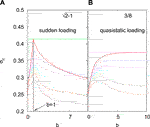FIGURE 13. The phase diagram in the $b-{\sigma }_{c}$ plane (b represents the anisotropy in the load redistribution process) is shown for (a) discrete step and (b) quasi-static loading for various fractional errors in the knowledge of the threshold values of the individual fibers (curves from top to below are for $e=0.0,0.1,0.2,0.3,0.4,0.5,0.75$). The upper bounds for both cases are shown, which are reached for $b=1$ (a) and $b\to \infty$ (b). From .

An important information in implementing the redistribution rule is the exact knowledge of the failure thresholds of all the surviving elements. This requirement may not be always fulfilled. Assuming that there is a (fractional) error e in the knowledge of the failure thresholds, numerical simulations show (see Figure 13) that the redistribution rule still gives better results than a uniform redistribution. Therefore, in situations where the load redistribution is controllable, the redistribution rule mentioned above gives the best possible outcome.

We would like to mention that cooperative dynamics appears in another class of fiber bundle models where fibers are treated as viscoelastic elements . The readers can go through  (appearing in the same research topic: The fiber bundle) for a review on viscoelastic fiber bundle models.

## 6 Summary and Conclusion

One can easily see that the fiber bundle model (FBM) introduced by Peirce  in 1926 as a model to understand the strength of composite materials is extremely elegant. As mentioned before, the model consists of a macroscopically large number of parallel fibers/springs with linear elastic behavior and of identical length. The breaking thresholds, however, are different for each fiber and are drawn from a probability distribution. All these fibers/springs hang from a rigid horizontal platform. The load on the bundle is applied at the lower horizontal platform. This lower platform has been assumed here to be rigid, implying that the stress or load share per surviving fibers/springs is equal, irrespective of how many fibers or springs might have broken (equal load sharing or ELS scheme). It may be mentioned that we have not discussed here the extensive studies on fiber bundle models with local load sharing (LLS) schemes, for which the readers may be advised to consult Refs. [1, 26], and the “impregnated fiber bundle” models for which the readers may be referred to Refs. [56, 57].

As discussed in this review, the failure dynamics of the FBM under the ELS scheme of load sharing have been analyzed for long, both analytically as well as numerically by several distinguished groups of investigators from engineering, physics, and applied mathematics. The results may be briefly summarized as follows: After introducing the model, we have described the dynamics of the equal load sharing (ELS) fiber bundle model in Section 2. Specifically, in this section, we discuss and summarize works (Refs. [1, 2, 4, 8, 28], see also [26, 40, 41]) related to the cooperative failure dynamics in the ELS fiber bundle model having a large number of fibers with different strength thresholds. We start this section by describing the force displacement relation (load curve) when the bundle is stretched by an amount x. The maximum point of this curve gives the strength of the whole bundle. One can easily derive the strength of the bundle for different fiber threshold distributions. We have chosen uniform and Weibull distributions as examples and derive bundles’ strength as critical displacement (xc) and critical force $\left({F}_{c}\right)$. Next, we describe how to formulate the dynamics of failure through a recursion relation in case of loading by discrete steps when fiber thresholds are uniformly distributed. The solution of the recursion relation at the fixed point gives some important information of the failure dynamics: Order parameter goes to zero following a power law as the applied stress values approach a critical value and both susceptibility and relaxation time diverge at the critical stress following well-defined power laws (see [4, 8, 42]). To check the universality of the failure dynamics, we choose different types of fiber strength distributions (linearly increasing) and derive the fixed-point solutions. The exponent values of the power laws for order parameter, susceptibility, and relaxation time variations are exactly the same as the model with a uniform distribution and therefore the failure dynamics in ELS fiber bundle model is universal. In addition, we present the exact solutions for pre- and post-critical relaxation behavior which we believe is one of the most important theoretical developments in this field. In the last part of this section, we present an analysis on the avalanche statistics for loading by a fixed amount. Such a loading scheme introduces a different mechanism for the avalanche sizes of simultaneous breaking of fibers. We discuss using analytical calculations that the exponent of the avalanche size distribution $\left(P\left(S\right)\right)$ for discrete loading would be $-3$, which is different ($-5/2$) from the same in the case of quasi-static loading situation .

In Section 3, we summarize some recent developments (Refs. [1116, 47, 49, 56]) in the cooperative dynamics of noise-induced failure in ELS fiber bundle models. In addition to applied stress, the noise factor plays a crucial role in triggering the failure of individual fibers. The trick here is how to define the failure probability of individual fibers as a function of applied/effective stress and the noise level. Normally, noise-level remains constant during the entire failure process, but the stress level increases gradually due to stress redistribution mechanism. The choice of the probability function should satisfy the fact that without the noise factor the noise-induced failure model must reproduce the classical failure scenario (discussed in Section 2). We start this section by presenting a noise-induced failure probability for individual fiber failure. The choice of stress and noise level dictates whether the system is in continuous breaking regime or in intermittent breaking regime. Through a mean-field argument, one can easily find out the phase diagram separating these two regimes (Eq. 50; Figure 6). Apparently, the continuous breaking regime is easy to analyze. For a homogeneous bundle, where all the fibers are identical (strengths are the same), one can write down the failure dynamics as a recursion relation (Eq. 53). The solution gives an exact estimate for the failure time (steps) as a function of applied stress (σ) and noise level (T) (Eq. 55). Simulation results show perfect agreement with the theoretical estimates (Figure 7). When we consider a strength distribution among the fibers in the model, it becomes extremely difficult to construct the recursion relation for the failure dynamics. One reason could be that during the failure process the strength distribution gets changed with time. However, the simulation results (Figure 8) for the failure time of heterogeneous bundles follow similar variation with applied stress and noise level with an extra noise factor (Eq. 56). Next, we discuss the other regime, that is, the intermittent failure regime where there is waiting time between the two failure phases. The distribution of the waiting time is the most important aspect in this regime. Simulation results on homogeneous and heterogeneous bundles show that the waiting time distribution follows a Gamma distribution (Eq. 57) and a data collapse confirms the universal nature of such distribution function (Figure 9). Surprisingly, waiting time distribution from earthquake time series (California catalog) seems to follow a similar Gamma distribution (Figure 10).

In Section 4, we have considered self-organized fracture front propagation in a fiber bundle model where the fracture front adjusts its size in a self-organized way to meet the increasing load on the bundle and several features of the self-organized dynamics can still be analyzed in a mean field way; see, for example, Figure 12 for the avalanche size distribution, which fits well with $D\left(S\right)\sim {S}^{-3/2}$.

As already mentioned (in Section 2), the universality class of the dynamics of fixed load increment during the ongoing dynamics of failure in the bundle (until its complete failure) will be different from that for the quasi-static (or weakest link failure type) loading during its dynamics. And, as discussed in Section 5, it is given by the Flory statistics for linear polymers, when fracture dynamics in the bundle is mapped to turbulence and one utilizes the Kolmogorov-type dispersion energy cascades . In particular, we already obtained (; see Eqs. 35 and 36) the order parameter exponent, $\beta =1/2=\gamma$, the susceptibility exponent. Employing the Rushbrooke scaling $2\beta +\gamma =\text{d}\nu$ (where ν denotes the correlation length exponent), we get $\text{d}\nu =3/2$ here in conformity with finite-size scaling results. As discussed in  (see also the discussions in Section 5), by mapping the avalanche size distribution (Eq. 47) to the Kolmogorov energy dispersion in turbulence (Eq. 67) and identifying S with the energy and inverse correlation length as the wave vector q, we got the upper critical dimension ${d}_{u}$ for FBM in the ELS scheme to be 6. This suggests that the correlation length exponent ν value here is $1/4$.

As discussed in this review, the absence of stress concentrations or fluctuations around the broken fibers allows mean-field-type statistical analysis in such equal load sharing fiber bundle models. This feature of the models helped major analytical studies for the breaking dynamics and also allowed precise comparisons with computer simulation results.

## Author Contributions

BKC made the initial plan for the review article. All the authors contributed equally in discussions and in the writing of the manuscript.

## Funding

This work was partly supported by the Research Council of Norway through its Centers of Excellence funding scheme, project number 262644.

## Conflict of Interest

The authors declare that the research was conducted in the absence of any commercial or financial relationships that could be construed as a potential conflict of interest.

## Acknowledgments

BKC is grateful to J. C. Bose Fellowship Grant for support.

## References

1. Pradhan S, Hansen A, Chakrabarti BK. Failure processes in elastic fiber bundles. Rev Mod Phys (2010) 82:499. doi:10.1103/RevModPhys.82.499

2. Pradhan S, Chakrabarti BK. Precursors of catastrophe in the Bak-Tang-Wiesenfeld, Manna, and random-fiber-bundle models of failure. Phys Rev E-Stat Nonlinear Soft Matter Phys (2002) 65:016113. doi:10.1103/PhysRevE.65.016113

3. Pradhan S, Bhattacharyya P, Chakrabarti BK. Dynamic critical behavior of failure and plastic deformation in the random fiber bundle model. Phys Rev E-Stat Nonlinear Soft Matter Phys (2002) 66:016116. doi:10.1103/PhysRevE.66.016116

4. Bhattacharyya P, Pradhan S, Chakrabarti BK. Phase transition in fiber bundle models with recursive dynamics. Phys Rev E-Stat Nonlinear Soft Matter Phys (2003) 67:046122. doi:10.1103/PhysRevE.67.046122

5. Peirce FT. Tensile tests for cottom yarns. “The weakest link” theorems on the strength of long and composite specimens. J Text Ind (1926) 17:355. doi:10.1080/19447027.1926.10599953

6. Hemmer PC, Hansen A. The distribution of simultaneous fiber failures in fiber bundles. ASME J Appl Mech (1992) 59:909. doi:10.1115/1.2894060

7. Pradhan S, Hansen A, Hemmer PC. Crossover behavior in burst avalanches: signature of imminent failure. Phys Rev Lett (2005) 95:125501. doi:10.1103/PhysRevLett.95.125501 |

8. Pradhan S, Hemmer PC. Relaxation dynamics in strained fiber bundles. Phys Rev E-Stat Nonlinear Soft Matter Phys (2007) 75:056112. doi:10.1103/PhysRevE.75.056112

9. Divakaran U, Dutta A. Effect of discontinuity in the threshold distribution on the critical behavior of a random fiber bundle. Phys Rev E-Stat Nonlinear Soft Matter Phys (2007) 75:011117. doi:10.1103/PhysRevE.75.011117

10. Lawn BR. Fracture of brittle solids. Cambridge, United Kingdom: Cambridge University Press (1993).

11. Coleman BD. Statistics and time dependence of mechanical breakdown in fibers. J Appl Phys (1958) 29:968. doi:10.1063/1.1723343

12. Scorretti R, Guarino A, Ciliberto S. Disorder enhances the effects of thermal noise in the fiber bundle model. Europhys Lett (2001) 55:626. doi:10.1209/epl/i2001-00462-x

13. Roux S. Thermally activated breakdown in the fiber-bundle model. Phys Rev E (2000) 62:6164. doi:10.1103/physreve.62.6164

14. Pradhan S, Chakrabarti BK. Failure due to fatigue in fiber bundles and solids. Phys Rev E - Stat Nonlinear Soft Matter Phys (2003) 67:046124. doi:10.1103/PhysRevE.67.046124

15. Reiweger I, Schweizer J, Dual J, Herrmann HJ. Modelling snow failure with a fibre bundle model. J Glaciol (2009) 55:997. doi:10.3189/002214309790794869

16. Cohen D, Lehmann P, Or D. Fiber bundle model for multiscale modeling of hydromechanical triggering of shallow landslides. Water Resour Res (2009) 45:W10436. doi:10.1029/2009WR007889

17. Pollen N, Simon A. Estimating the mechanical effects of riparian vegetation on stream bank stability using a fiber bundle model. Water Resour Res (2005) 41:W07025. doi:10.1029/2004WR003801

18. Pugno NM, Bosia F, Abdalrahman T. Hierarchical fiber bundle model to investigate the complex architectures of biological materials. Phys Rev E-Stat Nonlinear Soft Matter Phys (2012) 85:011903. doi:10.1103/PhysRevE.85.011903

19. Sornette D. Mean-field solution of a block-spring model of earthquakes. J Phys I France (1992) 2:2089. doi:10.1051/jp1:1992269

20. Daniels HE. The statistical theory of the strength of bundles of threads. Proc Roy Soc Ser A (1945) 183:243. doi:10.1098/rspa.1945.0011

21. Sornette D. Elasticity and failure of a set of elements loaded in parallel. J Phys Math Gen (1989) 22:L243.

22. Ising E. Beitrag zur theorie des ferromagnetismus. Z Phys (1925) 31:253. doi:10.1007/BF02980577

23. Harlow DG, Phoenix SL. Approximations for the strength distribution and size effect in an idealized lattice model of material breakdown. J Mech Phys Solid (1991) 39:173. doi:10.1016/0022-5096(91)90002-6

24. Gómez J, Iñiguez DAF, Pacheco AF. Solvable fracture model with local load transfer. Phys Rev Lett (1993) 71:380. doi:10.1103/PhysRevLett.71.380 |

25. Hidalgo RC, Kun YF, Herrmann HJ. Fracture model with variable range of interaction. Phys Rev E - Stat Nonlinear Soft Matter Phys (2002) 65:046148. doi:10.1103/PhysRevE.65.046148

26. Hansen A, Hemmer PC, Pradhan S. The fiber bundle model. Berlin, Germany: WileyVCH (2015).

27. Pradhan S, Chakrabarti BK. Introduction to critical phenomena through the fiber bundle model of fracture. Eur J Phys (2019) 40:014004. doi:10.1088/1361-6404/aaeb53

28. Biswas S, Chakrabarti BK. Flory-like statistics of fracture in the fiber bundle model as obtained via Kolmogorov dispersion for turbulence: a conjecture. Phys Rev E (2020) 102:012113. doi:10.1103/PhysRevE.102.012113 |

29. Flory PJ. Statistical mechanics of chain molecules. Hoboken, NJ: Wiley (1969)

30. Kolmogorov AN. Local structure of turbulence in an incompressible fluid at very high Renolds number. Dokl Akad Nauk SSSR (1941) 30:299. doi:10.1098/rspa.1991.0075

31. Biswas S, Ray P, Chakrabarti BK. Statistical physics of fracture, breakdown, and earthquake. Berlin, Germany: Wiley VCH (2015)

32. Kjellstadli JT. Burst distribution by asymptotic expansion in the equal load sharing fiber bundle model. Front Physiol (2019) 7:201. doi:10.3389/fphy.2019.00201

33. Pradhan S, Chandra A, Chakrabarti BK. Noise-induced rupture process: phase boundary and scaling of waiting time distribution. Phys Rev E - Stat Nonlinear Soft Matter Phys (2013) 88:012123. doi:10.1103/PhysRevE.88.012123

34. Petri A, Paparo G, Vespignani A, Alippi A, Costantini M. Experimental evidence for critical dynamics in microfracturing processes. Phys Rev Lett (1994) 73:3423. doi:10.1103/PhysRevLett.73.3423 |

35. Chakrabarti BK, Benguigui LG. Statistical physics of fracture and breakdown in disordered systems. Oxford, United Kingdom: Oxford University Press (1997)

36. Herrmann HJ, Roux S. Statistical models for the fracture of disordered media. Amsterdam, Netherlands: North-Holland (1990).

37.http://www.data.scec.org/ftp/catalogs/SHLK (Accessed February 1, 2005).

38. Corral A. Universal earthquake-occurrence jumps, correlations with time, and anomalous diffusion. Phys Rev Lett (2006) 97:178501. doi:10.1103/PhysRevLett.97.178501 |

39. Corral A. Local distributions and rate fluctuations in a unified scaling law for earthquakes. Phys Rev E - Stat Nonlinear Soft Matter Phys (2003) 68:035102. doi:10.1103/PhysRevE.68.035102

40. Bak P, Christensen K, Danon L, Scanlon T. Unified scaling law for earthquakes. Phys Rev Lett (2002) 88:178501. doi:10.1103/PhysRevLett.88.178501 |

41. Larkin AI, Ovchinnikov YN. Pinning in type II superconductors. J Low Temp Phys (1979) 34:409. doi:10.1007/BF00117160

42. Zapperi S, Cizeau P, Durin G, Stanley HE. Dynamics of a ferromagnetic domain wall: avalanches, depinning transition, and the Barkhausen effect. Phys Rev B (1998) 58:6353. doi:10.1103/PhysRevB.58.6353

43. Chevalier T, Talon L. Moving line model and avalanche statistics of Bingham fluid flow in porous media. Eur Phys J E Soft Matter (2015) 38:76. doi:10.1140/epje/i2015-15076-5 |

44. Biswas S, Chakrabarti BK. Self-organized dynamics in local load-sharing fiber bundle models. Phys Rev E-Stat Nonlinear Soft Matter Phys (2013) 88:042112. doi:10.1103/PhysRevE.88.042112

45. Hendrick M, Pradhan S, Hansen A. Mesoscopic description of the equal-load-sharing fiber bundle model. Phys Rev E (2018) 98:032117. doi:10.1103/PhysRevE.98.032117

46. Henkel M, Hinrichsen H, Lübeck S. Non-equilibrium phase transitions. In: Absorbing phase transitions, Vol. 1. Berlin, Germany: Springer (2008).

47. Basu A, Chakrabarti BK. Hydrodynamic descriptions for surface roughness in fracture front propagation. Philos Trans A Math Phys Eng Sci (2018) 377:20170387. doi:10.1098/rsta.2017.0387 |

48. Huang K. Lectures on statistical physics and protein folding. Singapore: World Scientific (2005).

CrossRef Full Text

49. Sinha S, Kjellstadli JT, Hansen A. Local load-sharing fiber bundle model in higher dimensions. Phys Rev E-Stat Nonlinear Soft Matter Phys (2015) 92:020401. doi:10.1103/PhysRevE.92.020401

50. Ma SK. Modern theory of critical phenomena. New York, NY: Taylor & Francis (1976).

51. Biswas S, Sen P. Maximizing the strength of fiber bundles under uniform loading. Phys Rev Lett (2015) 115:155501. doi:10.1103/PhysRevLett.115.155501 |

52. Hidalgo RC, Kun F, Herrmann HJ. Creep rupture of viscoelastic fiber bundles. Phys Rev E-Stat Nonlinear Soft Matter Phys (2002) 65:032502. doi:10.1103/PhysRevE.65.032502

53. Jagala EA. Creep rupture of materials: insights from a fiber bundle model with relaxation. Phys Rev E-Stat Nonlinear Soft Matter Phys (2011) 83:046119. doi:10.1103/PhysRevE.83.046119

54. Baxevanis T, Katsaounis T. Load capacity and rupture displacement in viscoelastic fiber bundles. Phys Rev E-Stat Nonlinear Soft Matter Phys (2007) 75:046104. doi:10.1103/PhysRevE.75.046104

55. Capelli A, Reiweger I, Schweizer J, Studying snow failure with fiber bundle models, Front Phys (2020) 8:236. doi:10.3389/fphy.2020.00236

56. Bunsell A, Gorbatikh L, Morton H, Pimenta S, Sinclair I, Spearing M, et al. Benchmarking of strength models for unidirectional composites under longitudinal tension. Composite A: Appl Sci Manufacturing (2018) 111:138. doi:10.1016/j.compositesa.2018.03.016

57. Breite C, Melnikov A, de Morais AB, Otero F, Mesquita F, Costa J, et al. Blind benchmarking of seven longitudinal tensile failure models for two virtual unidirectional composites. Compos Sci Technol (2021) 202:108555. doi:10.1016/j.compscitech.2020.108555

58. Pradhan S, Chakrabarti BK, Hansen A. Crossover behavior in a mixed-mode fiber bundle model. Phys Rev E - Stat Nonlinear Soft Matter Phys (2005) 71:036149. doi:10.1103/PhysRevE.71.036149

59. Roy S, Ray P. Critical behavior in fiber bundle model: a study on brittle to quasi-brittle transition. Europhys Lett (2015) 112:26004. doi:10.1209/0295-5075/112/26004

60. Roy C, Kundu S, Manna SS. Scaling forms for relaxation times of the fiber bundle model. Phys Rev E-Stat Nonlinear Soft Matter Phys (2013) 87:062137. doi:10.1103/PhysRevE.87.062137

61. Chakrabarti BK. A fiber bundle model of traffic jams. Physica A (2006) 377:162–166. doi:10.1016/j.physa.2006.05.003

62. Pahwa S, Scoglio C, Scala A. Abruptness of cascade failures in power grids. Sci Rep (2014) 4:3694. doi:10.1038/srep03694 |

63. Petri A, Pontuale G. Morphology and dynamics in SOC universality classes. J Stat Mech Theor Exp (2018) 6:063201. doi:10.1088/1742-5468/aac138

64. Pradhan S, Hansen A, Ray P. A renormalization group procedure for fiber bundle models. Front Physiol (2018) 6:65. doi:10.3389/fphy.2018.00065

65. Biswas S, Goehring L. Interface propagation in fiber bundles: local, mean-field and intermediate range dependent statistics. New J Phys (2016) 18:103048. doi:10.1088/1367-2630/18/10/103048

Keywords: fiber bundle model, dynamic cooperation, fixed-point solution, Universality, noise-induced failure dynamics, self-organization

Citation: Chakrabarti BK, Biswas S and Pradhan S (2021) Cooperative Dynamics in the Fiber Bundle Model. Front. Phys. 8:613392. doi: 10.3389/fphy.2020.613392

Received: 02 October 2020; Accepted: 23 December 2020;
Published: 15 February 2021.

Edited by:

Subhrangshu Sekhar Manna, S.N. Bose National Centre for Basic Sciences, India

Reviewed by:

Ferenc Kun, University of Debrecen, Hungary
Federico Bosia, Politecnico di Torin, Italy
Stepan Lomov, KU Leuven, Belgium

Copyright © 2021 Chakrabarti, Biswas and Pradhan. This is an open-access article distributed under the terms of the Creative Commons Attribution License (CC BY). The use, distribution or reproduction in other forums is permitted, provided the original author(s) and the copyright owner(s) are credited and that the original publication in this journal is cited, in accordance with accepted academic practice. No use, distribution or reproduction is permitted which does not comply with these terms.